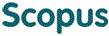### BROWSE

13 632

Cited 0 times in교합면과 Frankfort - mandibular plane angle 및 전치부 피개와의 관계에 대한 연구

Other Titles
(A) study of the occlusal plane as related to the frankfort - mandibular plane angle and incisal overlap
Authors
권소봉
Issue Date
1981
Description
치의학과/석사
Abstract
[한글]

저자는 1981년 현재 연세대학교 재학중인 남학생으로 비교적 정상 자연치열을 가진 80명을 조사표본으로하여, FMA (Frankfort-mandibular plane angle), 교합면 경사도, 전치부 수평, 수직피개도 및 교합만곡반경 간의 상관관계를 알고자 조사 분석하여 다음과 같

은 결론을 얻었다.

1. FMA와 교합면경사도와의 상관성은 단순 상관계수 0.711 (t =8.28, P< 0.01)로 높은 상관관계를 보였으며, 중회귀분석법에 의하면 교합면 경사도는 FMA만으로도 50.6%의 설명이 가능하였다.

2. 교합평면의 안이평면에 대한 평균 경사도는7.985°(S.E= 0.471, S.D.=3.475)로 나타났으며, FMA가 증가할 수록, 더욱 교합면경사도가 증가함을 보였다.

3. 정상자연치열에서 전 후 교합만곡반경의 평균치는 우측 3.24 inch (S.E.=0.161, S.D.=1.297), 좌측 3.33 inch(S.E=0.186, S.D.=1.496)로 나타났고, 중회귀분석결과, 전치부 수평수직피개도, FMA 및 교합면경사도의 교합만곡반경에 대한 설명도는 낮은 것으로 나타났다(좌측: 10.43%, 우측: 1.89%)

[영문]

This study was undertaken in order to stydy the occlusal plane as related to the FMA, occlusal curvature and incisal overlap.

Linear model measurement and roentgenographic cephalometric study were done on 80 young male strudents who had natural normal dentitions. The data were statistically analyzed.

the following results were obtained:

1. Correlation coefficient between the FMA and occlusal plane inclination was high in 0.711(t=8.28, p<0.01) and occlusal plane inclination could be explained by FMA in 50.6% by the multiple regression analysis.

2. The mean value of the occlusal plain inclination was 7.985˚, (S.E.=0.471, S.D.=3,475). The greater the FMA, the greater was the occlual plane inclination.

3. In the natural normal dentition, the mean value of the antero-posterior occlusal curve radius was 3.24 inch (Rt.S.E.=0.161, S.D.=1.297), 3.33inch (Lt.S.E.=0.186, S.D.=1,496) and by the multiple regression analysis, the coefficient of determination of incisal overlap, FMA and occlusal plane inclination to the occlusal curvature was low in value (Rt.;1.89%, Lt.;10.43%).
Full Text
https://ymlib.yonsei.ac.kr/catalog/search/book-detail/?cid=CAT000000004379
Files in This Item:
제한공개 원문입니다.
Appears in Collections:
2. College of Dentistry (치과대학) > Dept. of Advanced General Dentistry (통합치의학과) > 2. Thesis
URI
https://ir.ymlib.yonsei.ac.kr/handle/22282913/115102
사서에게 알리기